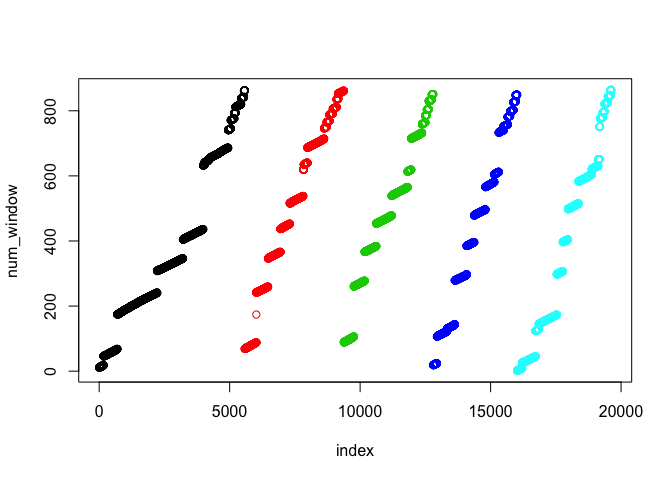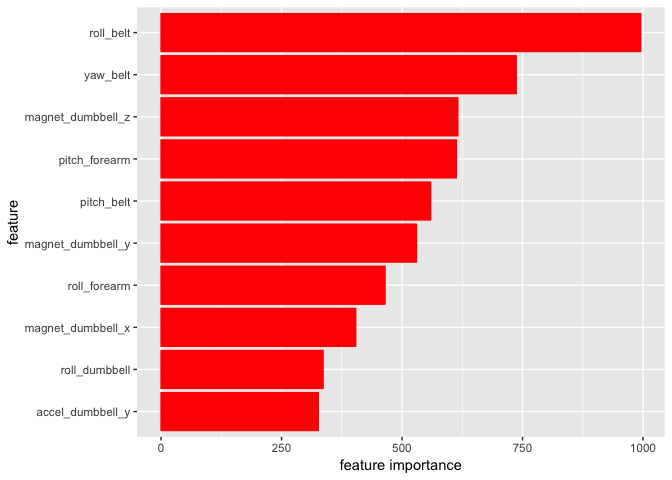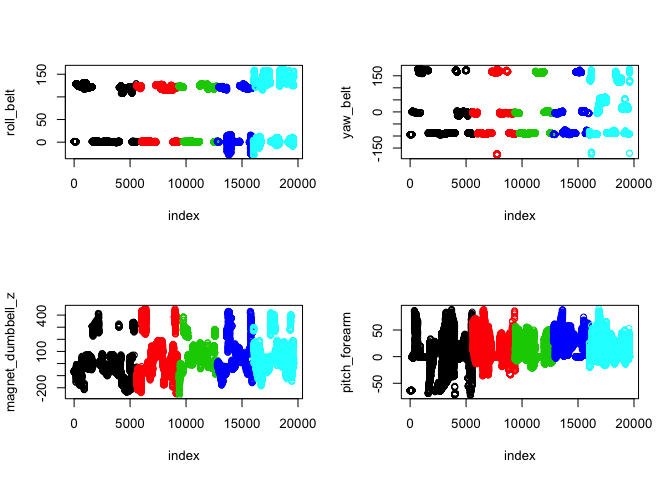## Introduction

A group of researchers asked participants to perform barbell lifts correctly and incorrectly in 5 different ways and recorded data from accelerometers on the belt, forearm, arm, and dumbell. We aim at creating a classification model for the 5 different types of motion. More information is available from the website here: http://web.archive.org/web/20161224072740/http:/groupware.les.inf.puc-rio.br/har (see the section on the Weight Lifting Exercise Dataset). The training data for this project are available here. The test data are available here.

df_train = read.csv('pml-training.csv')
df_test = read.csv('pml-testing.csv')

Regarding the missing values (NA), there are several columns with 19216 NA, which is the majority of the data. Therefore, we remove these columns

count_na_col = colSums(is.na(df_train))
df_train = df_train[, count_na_col == 0]
df_test = df_test[, count_na_col == 0] 

The following plot shows the relationship between num_window variable and the index and classes. This variable is likely generated by the experimentalists and should not be used.

plot(df_train$num_window, col = df_train$classe, xlab = 'index', ylab = 'num_window')Similar to new_window, X, user_name, timestamp, num_window are features irrelevant to the user’s motion.

df_train = df_train[, 8:ncol(df_train)]
df_test = df_test[, 8:ncol(df_test)]

Some numerical features appear to be factors so we first convert the factor variable to numeric. A number of them now contains mostly NA and are removed.

cols = seq(1, ncol(df_train) - 1)
df_train[,cols] = apply(df_train[,cols], 2, function(x) as.numeric(as.character(x)))
count_na_col = colSums(is.na(df_train))
df_train = df_train[, count_na_col == 0]
df_test = df_test[, count_na_col == 0] 

## Modelling

set.seed(42)
inTrain = createDataPartition(y = df_train$classe, p = 0.8, list = FALSE) training = df_train[inTrain, ] validation = df_train[-inTrain, ] base <- multinom(classe ~ ., training, trace = FALSE) pred = predict(base, validation, 'class') table(pred == validation$classe)
##
## FALSE  TRUE
##  1307  2616
tree_base = rpart(classe ~., training)
pred = predict(tree_base, validation, 'class')
table(pred == validation$classe) ## ## FALSE TRUE ## 954 2969 rf_base = randomForest(classe ~ ., training) pred = predict(rf_base, validation, 'class') table(pred == validation$classe)
##
## FALSE  TRUE
##    19  3904

The 19 misclassified examples are shown below and an accuracy of 99.5% is satisfactory. Thus we choose random forest for this task.

table(pred, validation$classe) ## ## pred A B C D E ## A 1116 3 0 0 0 ## B 0 752 1 0 0 ## C 0 4 683 9 1 ## D 0 0 0 633 0 ## E 0 0 0 1 720 We further verify the accuracy using cross-validation set.seed(123) folds <- createFolds(y = df_train$classe, k = 10, list = TRUE, returnTrain = FALSE)
acc_all = NULL
for (fold in folds){
validation = df_train[fold,]
rf = randomForest(classe ~ ., df_train[-fold,])
pred = predict(rf, validation)
acc_all = append(acc_all, mean(pred == validation$classe)) } acc_all ##  0.9959225 0.9989801 0.9938869 0.9969419 0.9974542 0.9979613 0.9964322 ##  0.9964304 0.9943963 0.9969419 The 95% confidence interval of the out-of-sample accuracy can be estimated from the 10-fold cross validation to be mean(acc_all) + c(-1, 1) * qt(0.975, df = 9) * sd(acc_all)/sqrt(10) ##  0.9954376 0.9976320 ## Random forest model We can visualise the feature importance by the mean decrease in Gini index of each feature and the top 10 are shown in the bar chart. They are of comparable importance and we can speculate that the prediction depends on a large number of features. imp = data.frame(name = names(training)[1: ncol(training) - 1], rf_base$importance)
imp = arrange(imp, desc(MeanDecreaseGini))
g <- ggplot(imp[1:10,], aes(x = reorder(name, MeanDecreaseGini), y = MeanDecreaseGini))
g <- g + geom_bar(stat='identity', col = 2, fill = 2) + coord_flip() +
labs(x = "feature", y = 'feature importance')
gThen the 4 most important features are plotted, colored according to the class label (A to E). It is likely that the features are continuous readings during the movement. At different stage of the motion, different feature may be important. For roll_belt, it is most discriminating for class E from the other classes and the pitch_forearm is important for differentiating class A. Given the performance of the classifier, we can conclude that random forest achieves accurate prediction at different stages of the motion by capturing the nonlinear interaction of the features.

par(mfrow = c(2,2))
plot(df_train$roll_belt, col = df_train$classe, xlab = 'index', ylab = 'roll_belt')
plot(df_train$yaw_belt, col = df_train$classe, xlab = 'index', ylab = 'yaw_belt')
plot(df_train$magnet_dumbbell_z, col = df_train$classe, xlab = 'index',
ylab = 'magnet_dumbbell_z')
plot(df_train$pitch_forearm, col = df_train$classe, xlab = 'index', ylab = 'pitch_forearm')Then we use this model to predict the class of the test dataset.

predict(rf_base, df_test, 'class')
##  1  2  3  4  5  6  7  8  9 10 11 12 13 14 15 16 17 18 19 20
##  B  A  B  A  A  E  D  B  A  A  B  C  B  A  E  E  A  B  B  B
## Levels: A B C D E

## Summary

In this study, we built classification models for the dumbell exercise using data from accelerometers on the belt, forearm, arm, and dumbell of 6 participants. Random forest model outperforms decision tree and multinomial regression and the 95% confidence interval of the accuracy is estimated using cross validation to be $$[0.995, 0.998]$$. It indicates that there are nonlinear relationship between features. By visualising the most important features from random forest model, the nonlinear relationship is confirmed.